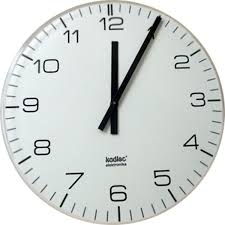# Denominator 16341

Express a fraction in the basic form of what part of the day represents 5 hours and 30 minutes. Write the result in the form of a reader, denominator.

a =  11
b =  48

### Step-by-step explanation:Did you find an error or inaccuracy? Feel free to write us. Thank you!

Tips for related online calculators
Need help calculating sum, simplifying, or multiplying fractions? Try our fraction calculator.
Do you want to convert time units like minutes to seconds?